# Some musings on the validation of Satow's Extraversion questionnaire

Measuring personality traits is one of (the?) bread-and-butter business of psychologists, at least for quantitatively oriented ones. Literally, thousand of psychometric questionnaires exits. Measures abound. Extroversion, part of the Big Five personality theory approach, is one of the most widely used, and extensively scrutinized questionnaire tapping into human personality.

One rather new, but quite often used questionnaire, is Satow’s (2012) B5T. The reason for the popularity of this instrument is that it runs under a CC-licence - in contrast to the old ducks, which coute chere. The B5T has undergone some psychometric scrutiny, and a number of results support the notion that it is a valid instrument.

Let’s look here into some aspects of validity of the instrument. More bluntly: Does the B5t (here: only extraversion) really measures extraversion? My point is rather not a judgement on this particular instrument, but more which checks can guide us to answer this question.

First, we do not know whether extraversion per se is of metric niveau; can the mind distinguish between infinitely many distinct yet equidistant steps on a imagined continuum? Surely this is a strong assumption. But let’s put that aside for now (as nearly everybody does not only for a moment but forever).

# External validity first

A primary check should be the external validity of the scale. When I say external validity, I mean e.g., whether the instrument is correlated with some variable it should be correlated with according to the theory we hold. That is to say, if some “obvious” fact is not identified by a measurement device, we would and should be unwilling to accept whether it really measures what it ought measure.

Note that reliability is not more than a premise or prerequisite for (external) validity. Thus, if we knew some measure is reliable (by standard procedures), we do not know whether it measures what it should measure. To the contrary, if there is some sound association with the right external measure, we are more confident (though maybe not satisfied).

## Data & packages

Let’s have a look at our extraversion (e) data. Here we go:

e <- read.csv("https://osf.io/meyhp/?action=download")


The DOI of this data is 10.17605/OSF.IO/4KGZH

We will need these packages:

library(tidyverse)

## Loading tidyverse: ggplot2
## Loading tidyverse: tibble
## Loading tidyverse: tidyr
## Loading tidyverse: readr
## Loading tidyverse: purrr
## Loading tidyverse: dplyr

## Conflicts with tidy packages ----------------------------------------------

## filter(): dplyr, stats
## lag():    dplyr, stats

library(htmlTable)
library(corrr)
library(psych)

##
## Attaching package: 'psych'

## The following objects are masked from 'package:ggplot2':
##
##     %+%, alpha

library(plotluck)


Note that recoding has already taken place, and a mean value has been computed; see here for details. The extraversion scale consists of 10 items, which 4 answer categories each. A codebook can be found here: https://osf.io/4kgzh/.

## Plotting means and frequencies

Let’s have a brief look at some stats as first step. Here are the histograms:

e %>%
select(i01:i10) %>%
gather %>%
ggplot +
aes(x = value) +
geom_bar()+
facet_wrap(~key, ncol = 4)

## Warning: Removed 24 rows containing non-finite values (stat_count).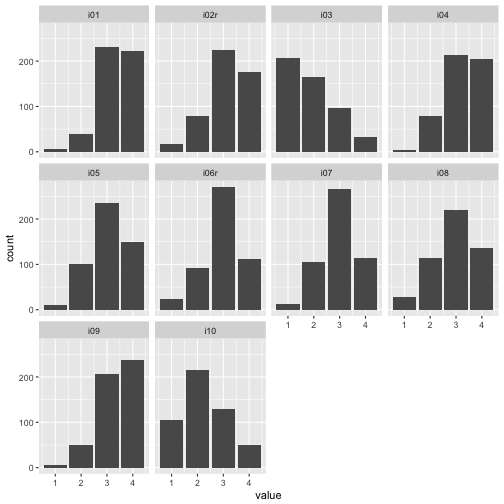Let’s save this dataframe because we will use it frequently:

e %>%
select(i01:i10) %>%
gather(key = item, value = value) -> e2


And means and SD:

e %>%
select(i01:i10) %>%
gather(key = item, value = value) %>%
group_by(item) %>%
summarise(item_mean = round(mean(value, na.rm = T), 2),
item_sd = round(sd(value, na.rm = T), 2)) -> e_summaries

htmlTable(e_summaries)

item item_mean item_sd
1 i01 3.34 0.68
2 i02r 3.12 0.8
3 i03 1.91 0.92
4 i04 3.24 0.73
5 i05 3.05 0.77
6 i06r 2.94 0.77
7 i07 2.97 0.73
8 i08 2.93 0.86
9 i09 3.35 0.71
10 i10 2.24 0.9

Maybe let’s plot that.

e_summaries %>%
mutate(mean = item_mean,
lower = mean - item_sd,
upper = mean + item_sd) %>%
select(-item_mean) -> e_summaries

e_summaries %>%
ggplot(aes(x = item, y = mean)) +
geom_errorbar(aes(ymin = lower,
ymax = upper),
color = "grey40") +
geom_point(color = "blue") +
labs(caption = "Error bars denote SDs")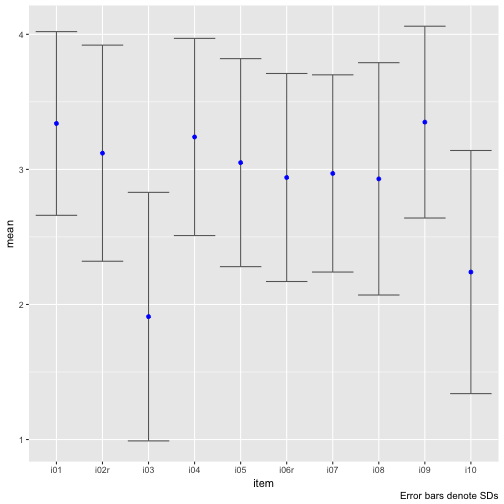Note that scale_y_continuous(limits = c(1,4)) will not work, because this method kicks out all values beyond those limits, which will screw up your error bars (I ran into that problem and it took me a while to see it).

Hm, there appear to types of items, one with a rather large mean, and one with a small mean. The SD appears to bear roughly equal.

Let’ sort the plot by the item means.

e_summaries %>%
ggplot(aes(x = reorder(item, mean), y = mean)) +
geom_errorbar(aes(ymin = lower,
ymax = upper),
color = "grey40") +
geom_point(color = "blue") +
labs(caption = "Error bars denote SDs")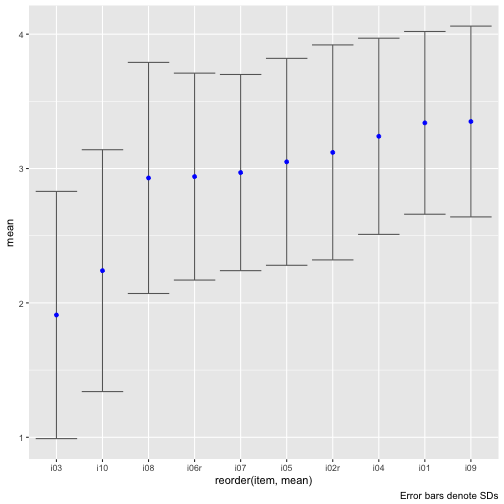Maybe plot stacked bar plots.

e2 %>%
na.omit %>%
count(item, value) %>%
ggplot +
aes(x = item, y = n, fill = value) +
geom_col() +
coord_flip()We see that at least the difficulty of the items ranged quite a bit. This last plot surely gives us the best impression on what’s going on. Note that real values have been depicted, not functions of them such as mean or sd.

For example, for nearly all items, mainly categories 3 and 4 were picked. So items were comparatively easy, which is not seldom. Maybe a finer grunalirity at the “bottom” would be of help to get more information.

## Correlation of means score with external criteria

OK, the correlation of the mean score with some external criteria surely is the central (first) idea to see how well the scale may have measured what it should measure.

We could assume that there is an association of extraversion and the number of Facebook friends.

cor(x = e$extra_mean, y = e$n_facebook_friends, use = "complete.obs") -> cor_mean_FB
cor_mean_FB

##  0.2558908


Hm, not really that much; however, in real life you have to be happy with non-perfect things…

There are a number of similar behavioral indicators in the data set besides number of FB friends. Let’s look at each in turn.

e %>%
select(extra_mean, time_conversation, n_party, n_hangover) %>%
correlate %>%
focus(extra_mean) %>%
mutate_if(is.numeric, funs(round), digits = 2) -> corr_emean_criteria

htmlTable(corr_emean_criteria)

rowname extra_mean
1 time_conversation -0.11
2 n_party 0.25
3 n_hangover 0.11

Basically not much, maybe n_party can be of interest.

Let’s plot a scatterplot.

e %>%
select(extra_mean, n_facebook_friends) %>%
qplot(x = extra_mean, y = n_facebook_friends, data = .) +
geom_smooth(method = "lm", se = FALSE) +
geom_label(x = 4, y = 2500, label = paste("r = ", round(cor_mean_FB, 2), sep = ""), hjust = 1)

## Warning: Removed 90 rows containing non-finite values (stat_smooth).

## Warning: Removed 90 rows containing missing values (geom_point).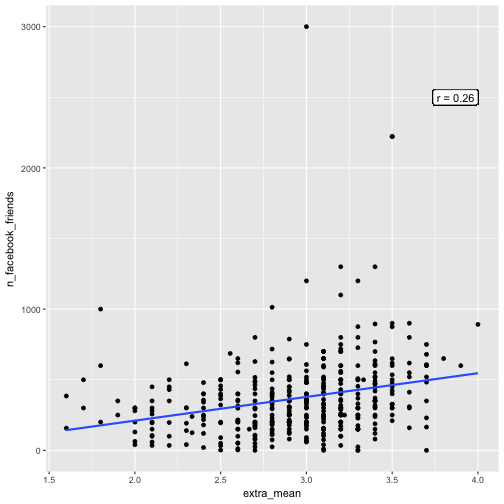Let’s skip the extreme high values of FB friends. The correlation appeared to got stronger:

e %>%
select(extra_mean, n_facebook_friends) %>%
filter(n_facebook_friends < 1500) %>%
correlate %>%
focus(extra_mean) -> cor_mean_FB_filtered
cor_mean_FB_filtered

## # A tibble: 1 × 2
##              rowname extra_mean
##                <chr>      <dbl>
## 1 n_facebook_friends  0.2774732

e %>%
select(extra_mean, n_facebook_friends) %>%
filter(n_facebook_friends < 1500) %>%
qplot(x = extra_mean, y = n_facebook_friends, data = .) +
geom_smooth(method = "lm", se = FALSE) +
geom_label(x = 4, y = 1000, label = round(cor_mean_FB_filtered$extra_mean, 2), hjust = 1)And now a scatterplot of extra_mean and n_party. e %>% select(extra_mean, n_party) %>% qplot(x = extra_mean, y = n_party, data = .) + geom_smooth(method = "lm", se = FALSE) + geom_label(x = 4, y = 120, label = paste("r = ", corr_emean_criteria$extra_mean, sep = ""), hjust = 1)

## Warning: Removed 18 rows containing non-finite values (stat_smooth).

## Warning: Removed 18 rows containing missing values (geom_point).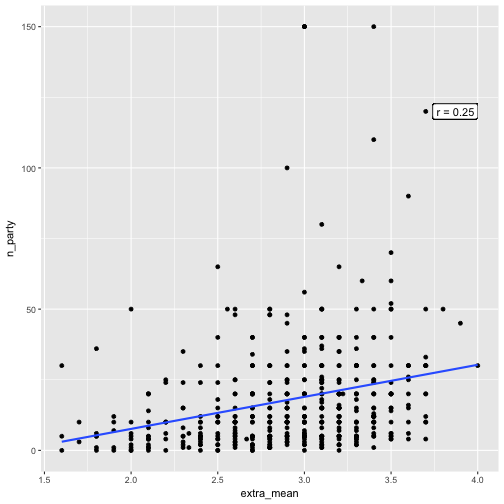## Correlation of extra_description with external criteria

Conventional psychometric knowledge holds that a psychometric ought to consist of several items. However, some renegades argue that “one-item wonder” can do an equally good job of predicting some external criteria. That’s no small claim, because if they do, there’s little to argue in favor of the longer, and thought more sophisticated methods in psychometry.

Of course, here we only play around a little, but let’s see what will happen:

• extra_description: One item self-description of extroversion
• extra_vignette: One item vignette (self-description) of extraversion

We start with extra_description:

e %>%
select(extra_description, time_conversation, n_party, n_hangover, n_facebook_friends, extra_mean) %>%
correlate %>%
focus(extra_description) %>%
ggplot(aes(x = rowname, extra_description)) +
geom_point() +
ggtitle("Correlation of extra_description with external criteria") +
ylab("correlation with extra_description")Let’s compare the correlation of extr_description with the external criteria to the correlation of extra_mean wit the external criteria.

e %>%
select(extra_description, time_conversation, n_party, n_hangover, n_facebook_friends) %>%
correlate %>%
focus(extra_description) %>%
mutate(predictor = "extra_description") %>%
rename(correlation = extra_description) -> corr_extra_description

e %>%
select(extra_mean, time_conversation, n_party, n_hangover, n_facebook_friends) %>%
correlate %>%
focus(extra_mean) %>%
mutate(predictor = "extra_mean") %>%
rename(correlation = extra_mean) -> corr_extra_mean

rbind(corr_extra_description, corr_extra_mean) -> corr_compare

corr_compare %>%
rename(criterion = rowname) -> corr_compare


Now let’s plot the difference in correlation strength for the two predictors of the external behavioral criteria.

corr_compare %>%
ggplot +
aes(x = criterion, y = correlation, color = predictor, fill = predictor) +
geom_rect(ymin = -0.1, ymax = 0.1, xmin = -10, xmax = 10, fill = "grey80", alpha = .2, color = NA) +
geom_point(aes(shape = predictor), size = 3) +
theme(legend.position = "bottom") +
coord_flip()Of interest, the correlation of number of Facebook friends is quite different for extra_description compared with the correlation of extra_mean. For the rest, the difference is not “too” strong.

## Assocation of extra_vignette with external criteria

extra_vignette needs some upfront preparation:

count(e, extra_vignette)

## # A tibble: 4 × 2
##          extra_vignette     n
##                  <fctr> <int>
## 1                         119
## 2         keine Antwort    65
## 3       passt insgesamt   238
## 4 passt insgesamt nicht    79

recode(e$extra_vignette, "keine Antwort" = "no_answer", "passt insgesamt" = "fit", "passt insgesamt nicht" = "no_fit", .default = NA_character_) -> e$extra_vignette

count(e, extra_vignette)

## # A tibble: 4 × 2
##   extra_vignette     n
##           <fctr> <int>
## 1      no_answer    65
## 2            fit   238
## 3         no_fit    79
## 4             NA   119


Let’s compute the mean value for each group of extra_vignette:

e %>%
select(extra_vignette, extra_description, time_conversation, n_party, n_hangover, n_facebook_friends, extra_mean) %>%
filter(extra_vignette %in% c("fit", "no_fit")) %>%
group_by(extra_vignette) %>%
summarise_all(funs(mean), na.rm = TRUE) %>%
mutate_if(is.numeric, funs(round), digits = 1) -> comp_extra_vignette

comp_extra_vignette %>%
htmlTable

extra_vignette extra_description time_conversation n_party n_hangover n_facebook_friends extra_mean
1 fit 2.7 12.1 20.7 11.2 417 3
2 no_fit 4 23.7 13.5 6.7 274.7 2.6

Let’s plot that too.

comp_extra_vignette %>%
gather(key = variable, value = value, -extra_vignette) %>%
ggplot +
aes(x = variable, y = value, color = extra_vignette, fill = extra_vignette) +
geom_point() +
scale_y_log10() +
coord_flip() +
labs(y = "value [log10]")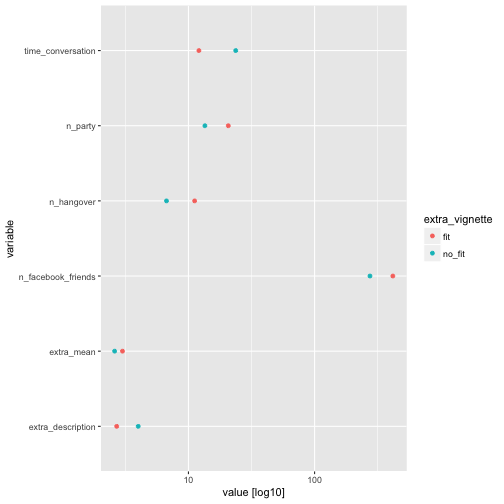We see that the fit dot is sometimes at a higher value (to the right) compared to the no_fit dot, which matches expectations. But this is, as can be seen, not always the case. Of interest, extra_mean shows small differences only.

OK, but what’s a big difference? Let’s compute the CLES measure of effect size for each criterion (here column of the table above) with extra_vignette as grouping variable.

First we prepare the data:

library(compute.es)

dplyr::count(e, extra_vignette)

e %>%
select(time_conversation, n_party, n_hangover, n_facebook_friends, extra_mean, extra_description, extra_vignette) %>%
filter(extra_vignette %in% c("fit", "no_fit")) %>%
na.omit() -> e3

e3 %>%
select(-extra_vignette) %>%
map(~t.test(. ~ e3$extra_vignette)) %>% map(~compute.es::tes(.$statistic,
n.1 = nrow(dplyr::filter(e, extra_vignette == "fit")),
n.2 = nrow(dplyr::filter(e, extra_vignette == "no_fit")))) %>%
map(~do.call(rbind, .)) %>%
as.data.frame %>%
t %>%
data.frame %>%
rownames_to_column %>%
rename(outcomes = rowname) ->
extra_effsize


Then we plot ‘em.

extra_effsize %>%
dplyr::select(outcomes, cl.d) %>%
mutate(sign = ifelse(cl.d > 50, "+", "-")) %>%
ggplot(aes(x = reorder(outcomes, cl.d), y = cl.d, color = sign)) +
geom_hline(yintercept = 50, alpha = .4) +
geom_point(aes(shape = sign)) +
coord_flip() +
ylab("effect size (Common Language Effect Size)") +
xlab("outcome variables") +
labs(title = "CLES plot for differences between groups of 'extra-vignette",
caption = "positive value are in favor of group 'fit'") -> CLES_plot

CLES_plot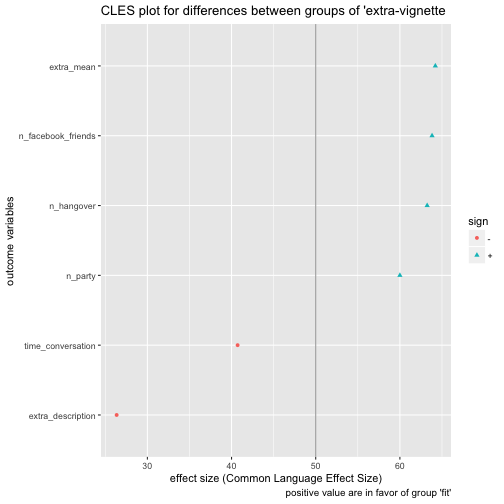Pooh, quite some fuzz. See this post for some explanation on what we have just done.

Now, what do we see? extra_description went plain wrong; extra_mean captures some information. We should note that some overcertainty may creep in here (and elsewhere). Our estimates are based on sample, not population values. So it is doubtful whether we can infer that futures samples (all we are interested in) will behave as predicted (here).

# Reliability

The only type of reliability we can compute is internal consistency, for we have no second measurement. It should be stressed that even if high reliability is given we do not know whether the measurement measured what we hope for. If reliability is not high, then we do know that our measurement failed. So reliability can be seen as necessary, but not sufficient, for gaining confidence in an instrument. That’s why validity (often read as correlation) is more telling: If association is there, we probably know what we wanted to know. Our measurement device as expected.

e %>%
select(i01:i10) %>%
psych::alpha()

##
## Reliability analysis
## Call: psych::alpha(x = .)
##
##   raw_alpha std.alpha G6(smc) average_r S/N   ase mean   sd
##       0.78      0.79    0.81      0.28 3.9 0.015  2.9 0.46
##
##  lower alpha upper     95% confidence boundaries
## 0.75 0.78 0.81
##
##  Reliability if an item is dropped:
##      raw_alpha std.alpha G6(smc) average_r S/N alpha se
## i01       0.75      0.76    0.77      0.26 3.2    0.017
## i02r      0.76      0.77    0.78      0.27 3.4    0.016
## i03       0.81      0.81    0.82      0.33 4.4    0.013
## i04       0.75      0.76    0.77      0.26 3.2    0.017
## i05       0.75      0.76    0.77      0.26 3.2    0.017
## i06r      0.76      0.77    0.78      0.27 3.4    0.016
## i07       0.76      0.77    0.79      0.27 3.4    0.016
## i08       0.76      0.78    0.79      0.28 3.5    0.016
## i09       0.77      0.78    0.79      0.28 3.6    0.016
## i10       0.78      0.79    0.80      0.30 3.8    0.015
##
##  Item statistics
##        n raw.r std.r r.cor r.drop mean   sd
## i01  499  0.69  0.71  0.68   0.60  3.3 0.68
## i02r 498  0.63  0.63  0.59   0.50  3.1 0.80
## i03  500  0.33  0.30  0.16   0.14  1.9 0.92
## i04  498  0.68  0.69  0.67   0.57  3.2 0.73
## i05  498  0.69  0.70  0.68   0.59  3.1 0.77
## i06r 499  0.62  0.62  0.57   0.50  2.9 0.77
## i07  498  0.62  0.62  0.57   0.50  3.0 0.73
## i08  499  0.61  0.60  0.53   0.47  2.9 0.86
## i09  499  0.55  0.57  0.49   0.43  3.4 0.71
## i10  498  0.51  0.49  0.39   0.34  2.2 0.90
##
## Non missing response frequency for each item
##         1    2    3    4 miss
## i01  0.01 0.08 0.46 0.45 0.00
## i02r 0.04 0.16 0.45 0.35 0.01
## i03  0.41 0.33 0.19 0.06 0.00
## i04  0.01 0.16 0.43 0.41 0.01
## i05  0.02 0.20 0.47 0.30 0.01
## i06r 0.05 0.18 0.54 0.22 0.00
## i07  0.02 0.21 0.54 0.23 0.01
## i08  0.06 0.23 0.44 0.27 0.00
## i09  0.01 0.10 0.41 0.47 0.00
## i10  0.21 0.43 0.26 0.10 0.01


Internal consistency, as an intuition, can be understood as something like the mean item inter correlation (the numbers are different, but the idea is similar). Note that Alpha is also a function of the number of items (the higher the number of items, the larger Alpha will be, not a nice property). And, even if Alpha is high that is no sufficient evidence that the data is unidimensional.

With the help of the manual of psych let’s dissect the output:

• raw_alpha: That’s the typical alpha you might expect (based on the covariances)
• average_r: Mean inter-item correlation
• S/N: signal-noise-ration (see manual for details)
• se: standard error (precision of estimation in Frequentist theory)
• rar.r: “The correlation of each item with the total score, not corrected for item overlap.”
• std.r: “The correlation of each item with the total score (not corrected for item overlap) if the items were all standardized”
• r.cor: “Item whole correlation corrected for item overlap and scale reliability”
• r.drop: “Item whole correlation for this item against the scale without this item”

Here’s some input on how to work with this function.

In sum, we may conclude that the internal consistency is good; item i03 appearing somewhat problematic as internal consistency would be higher without that guy.

Remember that i03 had the lowest mean? Fits together.

# Validity, next step

Let’s go back to some “validity” concerns, as this step is more important than reliability.

## Single-item external validity

Let’s compute the correlation of each item with a given external criterion. If the correlation of a single item is not worse than the total score, ~we~ the item score, that is the instrument is in trouble.

e %>%
select(i01:i10, n_facebook_friends) %>%
correlate %>%
focus(n_facebook_friends) %>%
mutate_if(is.numeric, round, digits = 2) %>%
rename(item = rowname, cor_with_n_FB_friends = n_facebook_friends) %>%
ggplot +
aes(x = reorder(item, cor_with_n_FB_friends), y = cor_with_n_FB_friends) +
geom_point() +
xlab("item") +
geom_hline(yintercept = cor_mean_FB, linetype = "dashed") +
geom_label(x = 5, y = cor_mean_FB, label = "correlation of mean score")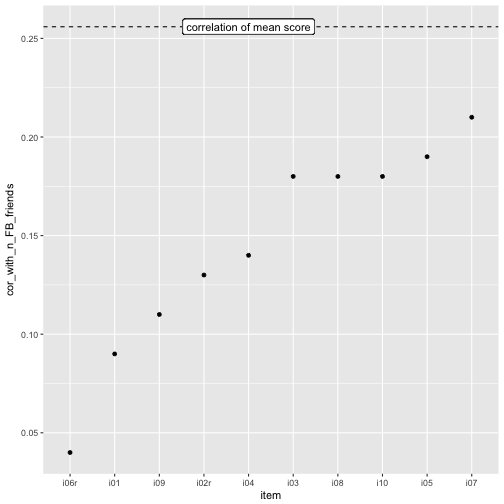OK, the mean score is “somewhat” better than the most predictive item, i07, but really really not much.

## Dichotomization

I know, some will say “don’t to that, my Goodness! Power!”, yes that’s true, but hey, let’s loose some power! After all, we are being more conservative, in a way. What do we get for this price? A quite tangible explanation. More precisely, let’s median split extra_mean and n_facebook_friends.

e %>%
select(extra_mean, n_facebook_friends) %>%
mutate(e_bin = ntile(extra_mean, 2),
n_fb_bin = ntile(n_facebook_friends, 2)) %>%
na.omit %>%
plotluck(e_bin ~ n_fb_bin)Hm, at least some association seem to be present.

## Non-linear association of extraversion with FB friends

We could apply different model, not only the linear model (ie., correlation) to discern possible patterns between the variables. This is an interesting field, but not my goal for today. Let’s dive here some other day; some time ago we have used some “machine learning” methods for this purpose in this paper.

## Finer categorial analysis

The idea of the dichotomization can be put to level of finer granularity. Why 2 buckets (bins)? Why not some more? This would give us a more precise picture. Additionally, it may argued that no measurement device exist with infinite granularity – which is posited by continuous variables (see Brigg’s book on that).

Of course, many ways exist to bin a metric variable into some discrete buckets including Sturges’ rule, or Freedman’s rule (see here for some explanation). But let’s go easy, and pick - arbitrarily - ten buckets for each variable of equal width (not of equal n).

e %>%
select(extra_mean, n_facebook_friends) %>%
na.omit %>%
mutate_all(cut_interval, n = 10) %>%
count(extra_mean, n_facebook_friends) %>%
ggplot(aes(x = extra_mean, y = n_facebook_friends, fill = n)) +
geom_bin2d()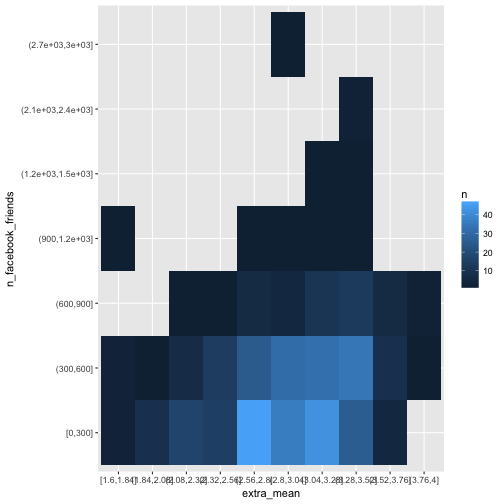One problem with this method here is that empty buckets are silently dropped which renders the procedure fruitless for our purpose.

Let’s try differently, and let’s kick out extreme values.

e %>%
select(extra_mean, n_facebook_friends) %>%
na.omit %>%
filter(n_facebook_friends < 1500) %>%
mutate_all(ntile, n = 10) %>%
count(extra_mean, n_facebook_friends) %>%
ggplot(aes(x = factor(extra_mean), y = factor(n_facebook_friends), fill = n)) +
geom_bin2d(aes(fill = n))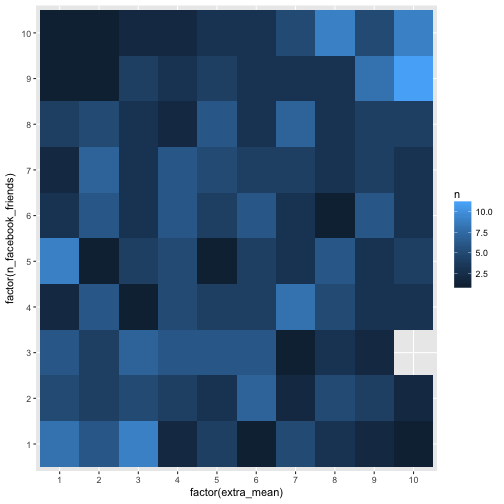Ah, that’s way better. Well, we do not see much, but the (absolute) numbers are not equal among the buckets.

 But even better would be if we could devise the probability of $y_n$ given $x_n$. More verbose, if the probability to have a 1 in X given Y is, say, 5 equals the probability $$p(X=2 Y=5)$$, then we will say that these two events are not related, or, more precisely, independent.

Let’s try it this way:

e %>%
select(extra_mean, n_facebook_friends) %>%
na.omit %>%
filter(n_facebook_friends < 1500) %>%
mutate_all(ntile, n = 10) %>%
count(n_facebook_friends, extra_mean) %>%
group_by(n_facebook_friends) %>%
mutate(prop = n/sum(n)) %>%
ggplot(aes(x = factor(extra_mean), y = factor(n_facebook_friends), fill = prop)) +
geom_bin2d(aes(fill = prop))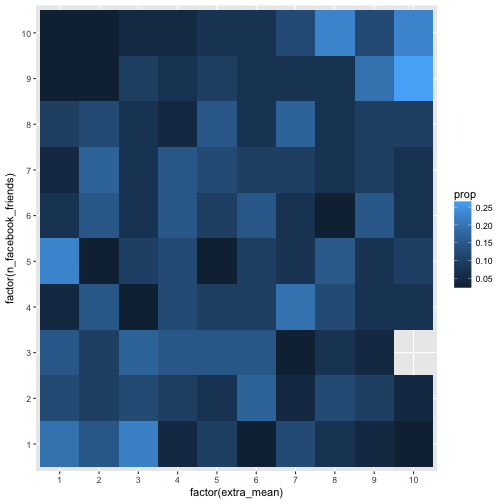Here we summed up rowwise. Let’s compare to what happens if we sum up colwise.

e %>%
select(extra_mean, n_facebook_friends) %>%
na.omit %>%
filter(n_facebook_friends < 1500) %>%
mutate_all(ntile, n = 10) %>%
count(extra_mean, n_facebook_friends) %>%
group_by(extra_mean) %>%
mutate(prop = n/sum(n)) %>%
ggplot(aes(x = factor(extra_mean), y = factor(n_facebook_friends), fill = prop)) +
geom_bin2d(aes(fill = prop))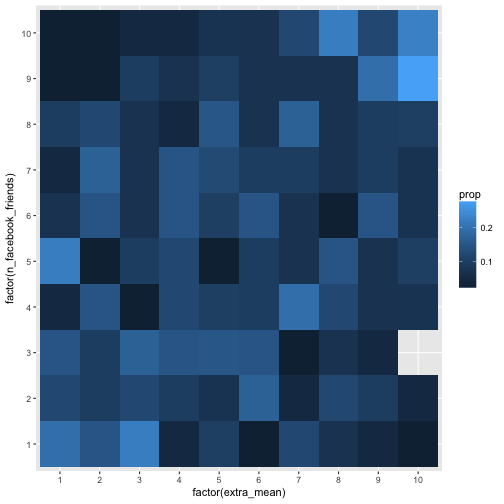Hm, maybe the association is too weak to see in that many buckets. Let’s reduce the bucket number by factor 2 (5 buckets), and check the picture again.

e %>%
select(extra_mean, n_facebook_friends) %>%
na.omit %>%
filter(n_facebook_friends < 1500) %>%
mutate_all(ntile, n = 5) %>%
count(extra_mean, n_facebook_friends) %>%
group_by(extra_mean) %>%
mutate(prop = n/sum(n)) %>%
ggplot(aes(x = factor(extra_mean), y = factor(n_facebook_friends), fill = prop)) +
geom_bin2d(aes(fill = prop))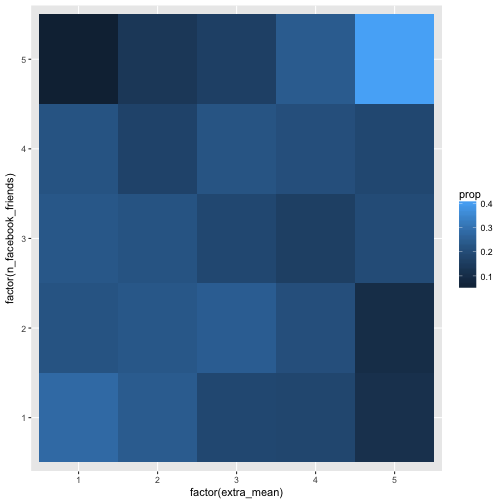This level of granularity seems more in place. Some association can be eyeballed. The conditional probabilities (p(Y=y X=x)) are not equal, thus, we can say, some dependencies exist. Note that this analyses is in a way more concrete than the correlation. Why? For two reasons. First, we speak on real observations, not on abstract parameters such as $r$ (see James Grice’ idea on Observation Oriented Modeling). Second, we do not shrunk down the information to one number only, but too much more (here 25=5x5), this amount of information can easily be recognized by the eye.

There is a wealth on categorial data analysis tools available, notable Agresti’s work. Thus, much more could be said and done.

## CLES

The “Common language effect size” (CLES) is an interesting approach to put effect sizes, such as $r$, to a more tangible language. Basically, CLES tells us, if we were to draw two observations, one of each of two group (here: high vs. low extraversion), what’s the probability that the observation from the “experimental” group (or reference group, more generally) will show higher value in the outcome variable? Note that it is note the same as a Bayesian predictive distribution.

An R function for that purpose exists, but see this great post for more in depth info.

# Final thoughts

The central idea of this post was to see whether the mean score is more predictive to some criterion than single items. I argue that validity is more important than reliability, albeit reliability (Cronbach’s Alpha) is typically reported in (applied) papers, but validity to a lesser degree.

Second, we explored (a bit) the notion whether the association is better captured in several numbers, and not only in one, as is done by the correlation value. This idea is based on the definition of independence in probability theory.

We did not dive here in factor analytic waters, nor did we discussed Item Response Theory, amongst other ideas left out. These ideas are worthwhile certainly, but beyond the primary aims of this post.

# Related work

There’s a lot on scale validation on the internet; see here for an example. For skepticism on psychometric scale, and for advocates of single item scales, see here, or here, and here for a broader discussion.

# SessionInfo

sessionInfo()

## R version 3.3.2 (2016-10-31)
## Platform: x86_64-apple-darwin13.4.0 (64-bit)
## Running under: macOS Sierra 10.12.1
##
## locale:
##  en_US.UTF-8/en_US.UTF-8/en_US.UTF-8/C/en_US.UTF-8/en_US.UTF-8
##
## attached base packages:
##  stats     graphics  grDevices utils     datasets  methods   base
##
## other attached packages:
##   compute.es_0.2-4 plotluck_1.1.0   psych_1.6.9      corrr_0.2.1
##   htmlTable_1.7    dplyr_0.5.0      purrr_0.2.2.9000 readr_1.0.0
##   tidyr_0.6.0      tibble_1.2       ggplot2_2.2.0    tidyverse_1.0.0
##  knitr_1.15
##
## loaded via a namespace (and not attached):
##   Rcpp_0.12.7         magrittr_1.5        mnormt_1.5-5
##   munsell_0.4.3       colorspace_1.3-0    R6_2.2.0
##   highr_0.6           stringr_1.1.0       plyr_1.8.4
##  tools_3.3.2         parallel_3.3.2      grid_3.3.2
##  gtable_0.2.0        DBI_0.5-1           htmltools_0.3.5
##  yaml_2.1.14         lazyeval_0.2.0.9000 rprojroot_1.1
##  digest_0.6.10       assertthat_0.1      RColorBrewer_1.1-2
##  evaluate_0.10       rmarkdown_1.1.9016  labeling_0.3
##  stringi_1.1.2       scales_0.4.1        backports_1.0.4
##  foreign_0.8-67


# Preparing survey results data

Analyzing survey results is a frequent endeavor (for some including me). Let’s not think about arguments whether and when surveys are useful or not (for some recent criticism see Briggs’ book).

Typically, respondents circle some option ranging from “don’t agree at all” to “completely agree” for each question (or “item”). Typically, four to six boxes are given where one is expected to tick one.

In this tutorial, I will discuss some typical steps to prepare the data for subsequent analyses. The goal is that we have the dataset ready for analyzing with basic preparations (eg. recoding of reversed variables) already done.

Some needed packages.

library(tidyverse)
library(forcats)  # recoding
library(psych)  # testing for internal consistency


# Prepare data

So, first, let’s load some data. That’s a data set of a survey on extraversion. Folks were asked a bunch of questions tapping at their “psychometric extraversion”, and some related behavior, that is, behavior supposed to be related such as “number of Facebook friends”, “how often at a party” etc. Note that college students form the sample.

Data are available only (free as in free and free as in beer).

data_raw <- read.csv("https://sebastiansauer.github.io/data/extra_raw_WS16.csv")

data <- data_raw  # backup just in case


Here’s the DOI of this data: 10.17605/OSF.IO/4KGZH.

OK, we got ‘em; a dataset of dimension 501, 24. Let’s glimpse at the data:

glimpse(data)

## Observations: 501
## Variables: 24
## $Zeitstempel <fctr> ... ##$ Bitte.geben.Sie.Ihren.dreistellen.anonymen.Code.ein..1...Anfangsbuchstabe.des.Vornamens.Ihres.Vaters..2...Anfangsbuchstabe.des.Mädchennamens.Ihrer.Mutter..3..Anfangsbuchstabe.Ihres.Geburstsorts.                                                                                                                                                                                                                                                                                                                                                                                                                                                                                                                                                                                     <fctr> ...
## $Ich.bin.gerne.mit.anderen.Menschen.zusammen. <int> ... ##$ Ich.bin.ein.Einzelgänger.....                                                                                                                                                                                                                                                                                                                                                                                                                                                                                                                                                                                                                                                                                                                                                          <int> ...
## $Ich.bin.in.vielen.Vereinen.aktiv. <int> ... ##$ Ich.bin.ein.gesprächiger.und.kommunikativer.Mensch.                                                                                                                                                                                                                                                                                                                                                                                                                                                                                                                                                                                                                                                                                                                                    <int> ...
## $Ich.bin.sehr.kontaktfreudig. <int> ... ##$ Im.Grunde.bin.ich.oft.lieber.für.mich.allein.....                                                                                                                                                                                                                                                                                                                                                                                                                                                                                                                                                                                                                                                                                                                                      <int> ...
## $Ich.kann.schnell.gute.Stimmung.verbreiten. <int> ... ##$ Ich.gehe.gerne.auf.Partys.                                                                                                                                                                                                                                                                                                                                                                                                                                                                                                                                                                                                                                                                                                                                                             <int> ...
## $Ich.bin.unternehmungslustig. <int> ... ##$ Ich.stehe.gerne.im.Mittelpunkt.                                                                                                                                                                                                                                                                                                                                                                                                                                                                                                                                                                                                                                                                                                                                                        <int> ...
## $Wie.viele.Facebook.Freunde..Kontakte..haben.Sie..wenn.Sie.nicht.bei.Facebook.sind..bitte.LEER.lassen.. <int> ... ##$ Wie.viele..Kater...überreichlicher.Alkoholkonsum..hatten.Sie.in.den.letzten12.Monaten.                                                                                                                                                                                                                                                                                                                                                                                                                                                                                                                                                                                                                                                                                                 <fctr> ...
## $Wie.alt.sind.Sie. <int> ... ##$ Bitte.geben.Sie.Ihr.Geschlecht.an.                                                                                                                                                                                                                                                                                                                                                                                                                                                                                                                                                                                                                                                                                                                                                     <fctr> ...
## $Ich.würde.sagen..ich.bin.extrovertiert. <int> ... ##$ Sie.gehen.alleine.auf.eine.Party..Nach.wie.viel.Minuten.sind.Sie.im.Gespräch.                                                                                                                                                                                                                                                                                                                                                                                                                                                                                                                                                                                                                                                                                                          <fctr> ...
## $Es.wird.ein.Mitarbeiter..m.w..für.eine.Präsentation..Messe..gesucht..Melden.Sie.sich.freiwillig. <fctr> ... ##$ Wie.häufig.waren.Sie.in.den.letzten.12.Monaten.auf.einer.Party.                                                                                                                                                                                                                                                                                                                                                                                                                                                                                                                                                                                                                                                                                                                        <fctr> ...
## $Wie.oft.haben.Sie.Kundenkontakt. <fctr> ... ##$ Passt.die.folgende.Beschreibung.auf.Sie...........Eine.extravertierte.Person.ist.jemand..der.seine.Energie.eher.nach.außen.richtet.und.weniger.in.die.innere.Welt.der.Gedanken..Ideen.oder.Vorstellungen..Daher.neigen.extravertierte.Menschen.dazu..in.neuen.Situationen.ohne.zu.zögern.sich.in.die.neue.Situationen.zu.begeben..Zum.Beispiel.würde.eine.extravertierte.Person..die.zum.ersten.Mal.einen.Yogakurs.besucht..sich.nicht.scheuen..direkt.bei.den.Übungen.mitzumachen..Oder.wenn.eine.extravertierte.Person.eine.Kneipe.zum.ersten.besucht..würde.sie.sich.nicht.unbehaglich.fühlen..Man.kann.daher.sagen..dass.extravertierte.Personen.als.aktiv.wahrgenommen.werden.und.sich.zu.Unternehmungen.hingezogen.fühlen..bei.denen.sie.mit.anderen.Personen.in.Kontakt.kommen. <fctr> ...
## $X <lgl> ... ##$ Wie.sehr.treffen.die.folgenden.Aussagen.auf.Sie.zu..Bitte.denken.Sie.dabei.nicht.an.spezifische.Situationen..sondern.ganz.allgemein..wie.sehr.die.Aussagen.Sie.selbst.in.den.meisten.Bereichen.und.Situationen.in.Ihrem.Leben.beschreiben.Allgemein.wirke.ich.tendenziell.eher.wie.eine.Person..die......                                                                                                                                                                                                                                                                                                                                                                                                                                                                              <int> ...


## Renaming

Looks like a jungle. Now what? Let’s start with renaming the columns (variables).

extra_colnames <- names(data)  # save names in this vector

names(data)[3:12] <- paste("i", formatC(1:10, width = 2, flag = "0"), sep = "")


There’s a saying that naming variables/objects in one of the hardest things in computer stuff. One time I renamed the columns from “i01” to “i26” or so. That’s different to the naming scheme we used now! This ambivalence can lead to confusion (happened to me). That’s why I now follow this rule:

• Renaming strange columns names -> use v01 to v25 (or how many columns you have)
• Renaming items of e.g. some questionnaire -> use 01 to i10 etc.

Now columns 3 to 12 are now named “i1”, “i2”, etc. These columns reflect the items of a extraversion questionnaire.

names(data) <- "timestamp"
names(data) <- "code"
names(data) <- "n_facebook_friends"
names(data) <- "n_hangover"
names(data) <- "age"
names(data) <- "sex"
names(data) <- "extra_single_item"
names(data) <- "time_conversation"
names(data) <- "presentation"
names(data) <- "n_party"
names(data) <- "clients"
names(data) <- "extra_vignette"
names(data) <- "extra_description"
data$X <- NULL  ## Recoding Importantly, two items are negatively coded; we need to recode them (ie., “yes” gets “no”, and vice versa). They are: extra_colnames[c(4, 8)]  ##  "Ich.bin.ein.Einzelgänger....." ##  "Im.Grunde.bin.ich.oft.lieber.für.mich.allein....."  Double check whether still nothing is recoded (I’ve run this code a couple of times already). identical(data$i02, data_raw[])

##  TRUE

identical(data$i06, data_raw[])  ##  TRUE  OK. rename(data, i02r = i02, i06r = i06) -> data data %>% mutate(i02r = recode(i02r, 1 = 4L, 2 = 3L, 3 = 2L, 4 = 1L), i06r = recode(i06r, 1 = 4L, 2 = 3L, 3 = 2L, 4 = 1L)) -> data  This may lead us too far afield, but let’s see whether the items correlate with each other, and each with the rest. We can compute Cronbach’s Alpha for that purpose data %>% select(i01:i10) %>% psych::alpha() %>% print  ## ## Reliability analysis ## Call: psych::alpha(x = .) ## ## raw_alpha std.alpha G6(smc) average_r S/N ase mean sd ## 0.78 0.79 0.81 0.28 3.9 0.015 2.9 0.46 ## ## lower alpha upper 95% confidence boundaries ## 0.75 0.78 0.81 ## ## Reliability if an item is dropped: ## raw_alpha std.alpha G6(smc) average_r S/N alpha se ## i01 0.75 0.76 0.77 0.26 3.2 0.017 ## i02r 0.76 0.77 0.78 0.27 3.4 0.016 ## i03 0.81 0.81 0.82 0.33 4.4 0.013 ## i04 0.75 0.76 0.77 0.26 3.2 0.017 ## i05 0.75 0.76 0.77 0.26 3.2 0.017 ## i06r 0.76 0.77 0.78 0.27 3.4 0.016 ## i07 0.76 0.77 0.79 0.27 3.4 0.016 ## i08 0.76 0.78 0.79 0.28 3.5 0.016 ## i09 0.77 0.78 0.79 0.28 3.6 0.016 ## i10 0.78 0.79 0.80 0.30 3.8 0.015 ## ## Item statistics ## n raw.r std.r r.cor r.drop mean sd ## i01 499 0.69 0.71 0.68 0.60 3.3 0.68 ## i02r 498 0.63 0.63 0.59 0.50 3.1 0.80 ## i03 500 0.33 0.30 0.16 0.14 1.9 0.92 ## i04 498 0.68 0.69 0.67 0.57 3.2 0.73 ## i05 498 0.69 0.70 0.68 0.59 3.1 0.77 ## i06r 499 0.62 0.62 0.57 0.50 2.9 0.77 ## i07 498 0.62 0.62 0.57 0.50 3.0 0.73 ## i08 499 0.61 0.60 0.53 0.47 2.9 0.86 ## i09 499 0.55 0.57 0.49 0.43 3.4 0.71 ## i10 498 0.51 0.49 0.39 0.34 2.2 0.90 ## ## Non missing response frequency for each item ## 1 2 3 4 miss ## i01 0.01 0.08 0.46 0.45 0.00 ## i02r 0.04 0.16 0.45 0.35 0.01 ## i03 0.41 0.33 0.19 0.06 0.00 ## i04 0.01 0.16 0.43 0.41 0.01 ## i05 0.02 0.20 0.47 0.30 0.01 ## i06r 0.05 0.18 0.54 0.22 0.00 ## i07 0.02 0.21 0.54 0.23 0.01 ## i08 0.06 0.23 0.44 0.27 0.00 ## i09 0.01 0.10 0.41 0.47 0.00 ## i10 0.21 0.43 0.26 0.10 0.01  Ok, looks good. I suspect there are rows with no values at, complete blank. Let’s compute the proportion of NAs per row. rowSums(is.na(data))  ##  1 1 1 1 1 1 1 1 1 2 1 1 1 1 1 1 1 2 1 1 1 1 1 ##  2 1 2 2 1 1 1 1 1 1 1 1 1 1 2 1 2 1 1 1 1 1 1 ##  1 1 2 1 1 3 1 1 1 1 1 1 1 1 1 1 1 1 1 1 1 1 1 ##  1 1 1 1 1 1 1 2 1 1 1 1 1 1 1 1 1 1 1 1 2 2 1 ##  1 2 1 1 1 2 2 1 1 2 1 1 3 1 1 1 1 2 1 2 1 1 1 ##  0 0 0 0 0 0 0 1 0 0 0 0 0 0 0 0 1 0 0 1 0 1 0 ##  1 0 0 1 0 0 0 0 0 0 1 0 0 0 0 0 0 0 0 1 0 0 2 ##  0 0 0 0 0 1 1 0 0 0 0 0 0 1 0 1 0 1 0 0 1 1 1 ##  1 0 3 0 2 0 0 0 0 0 0 1 1 0 0 0 0 1 1 0 0 0 0 ##  0 0 0 0 0 0 0 0 0 0 0 0 1 0 0 0 0 1 0 0 0 1 1 ##  1 1 0 1 1 0 1 1 0 0 0 1 0 0 0 0 0 0 0 0 0 0 0 ##  0 0 0 0 0 0 0 0 0 0 1 0 0 0 0 1 0 0 0 0 0 0 0 ##  0 1 0 1 0 0 0 1 0 0 0 0 0 0 0 0 0 0 0 1 0 0 0 ##  0 0 0 0 0 0 1 0 0 1 0 0 0 0 0 0 2 0 0 0 0 0 0 ##  1 0 0 1 0 0 0 0 0 0 0 1 0 0 0 0 0 0 1 1 0 0 0 ##  0 0 0 1 0 0 2 0 0 0 0 1 1 0 0 0 0 0 0 0 0 1 0 ##  0 0 1 0 0 0 0 0 0 0 1 1 0 0 0 0 1 0 0 0 0 0 1 ##  0 1 0 1 0 0 0 0 0 0 0 0 0 0 0 0 0 0 1 0 0 0 0 ##  0 0 0 0 0 0 0 0 0 0 1 1 0 0 0 0 0 0 0 1 0 0 0 ##  0 0 0 1 1 0 1 0 0 0 0 0 0 0 0 14 1 1 0 1 0 1 0 ##  0 0 0 0 0 11 0 0 0 1 0 0 0 1 1 0 1 1 0 0 0 0 0 ##  0 0 0 0 0 0 0 0 0 0 0 0 1 1 0 0 1 0  table(data$extra_description)

##
##   1   2   3   4   5   6   7   8  10
##  31 118  98  68  44  12   5   1   1

data %>%
mutate(prop_na_per_row = rowSums(is.na(data))/ncol(data)) -> data

count(data, prop_na_per_row)

## # A tibble: 6 × 2
##   prop_na_per_row     n
##             <dbl> <int>
## 1      0.00000000   304
## 2      0.04347826   171
## 3      0.08695652    21
## 4      0.13043478     3
## 5      0.47826087     1
## 6      0.60869565     1


## Rowwise means for survey etc.

Let’s compute the mean and the median extraversion.

data %>%
mutate(extra_mean = rowMeans(.[3:12], na.rm = TRUE),
extra_median = apply(.[3:12], 1, median, na.rm = TRUE)) -> data


## Cleaning data

The number of hangovers should be numeric, but it isn’t. Let’s see.

count(data, n_hangover) %>% kable

n_hangover n
13
0 98
1 59
10 38
100 1
106 4
12 18
15 10
153 1
18 1
2 63
20 14
200 1
24 8
25 5
28 1
3 42
30 11
35 1
4 25
40 9
48 2
5 38
50 6
6 15
7 2
70 2
8 7
80 1
9 2
98 1
ca. 18-23 1
Keinen 1

OK, let’s parse the numbers only; a typical problem in surveys is that respondent do not give numbers where you would like them to give numbers (some survey tools allow you to control what the respondent may put in the field).

data$n_hangover <- parse_number(data$n_hangover)

## Warning: 2 parsing failures.
## row col expected actual
## 132  -- a number
## 425  -- a number      .

data$time_conversation <- parse_number(data$time_conversation)

## Warning: 1 parsing failure.
## row col expected actual
## 153  -- a number

data$n_party <- parse_number(data$n_party)

## Warning: 1 parsing failure.
## row col expected actual
## 270  -- a number

# data$n_clients <- parse_number(data$clients)


For the item “how many clients do you see each weak”, things are more difficult, see:

data %>%
count(clients)

## # A tibble: 19 × 2
##                                        clients     n
##                                         <fctr> <int>
## 1                                                 51
## 2                                            0     3
## 3                                          100     1
## 4                              3 Mal pro Woche     1
## 5                                    gar nicht     1
## 6                            habe keine Kunden     1
## 7                            ich arbeite ncht      1
## 8                Ich habe keinen Kundenkontakt     1
## 9                   im Schnitt 1 Mal pro Monat    30
## 10 im Schnitt 1 Mal pro Quartal (oder weniger)    51
## 11                    im Schnitt 1 Mal pro Tag    50
## 12                  im Schnitt 1 Mal pro Woche    78
## 13                 im Schnitt mehrfach pro Tag   225
## 14                                      keinen     1
## 15                                         nie     2
## 16                                         Nie     1
## 17                                          Nö     1
## 18                      täglich zwischen 20-50     1
## 19    Telfonisch täglich, Face to Face 1xWoche     1


There are some values/words indicating that the respondent does not see any clients at all. Let’s recode them to “I am not having personal contact to clients” (or, shorter no).

data %>%
mutate(client_freq = recode(clients, "gar nicht" = "0",
"habe keine Kunden" = "0",
"keinen" = "0",
"Nie" = "0",
"Nö" = "0",
"Ich habe keinen Kundenkontakt" = "0",
"ich arbeite ncht " = "0",
"nie" = "0",
" " = "0")) -> data

data %>%
count(client_freq)

## # A tibble: 11 × 2
##                                    client_freq     n
##                                         <fctr> <int>
## 1                                                 51
## 2                                            0    12
## 3                                          100     1
## 4                              3 Mal pro Woche     1
## 5                   im Schnitt 1 Mal pro Monat    30
## 6  im Schnitt 1 Mal pro Quartal (oder weniger)    51
## 7                     im Schnitt 1 Mal pro Tag    50
## 8                   im Schnitt 1 Mal pro Woche    78
## 9                  im Schnitt mehrfach pro Tag   225
## 10                      täglich zwischen 20-50     1
## 11    Telfonisch täglich, Face to Face 1xWoche     1


Ok, looks good, but still a bit work left.

data %>%
mutate(client_freq = recode(client_freq, "100" = "3",
"im Schnitt 1 Mal pro Quartal (oder weniger)" = "1",
"im Schnitt 1 Mal pro Monat" = "2",
"im Schnitt 1 Mal pro Woche" = "3",
"im Schnitt 1 Mal pro Tag" = "4",
"im Schnitt mehrfach pro Tag" = "5",
"täglich zwischen 20-50" = "5",
"Telfonisch täglich, Face to Face 1xWoche" = "5",
"3 Mal pro Woche" = "4")) -> data

data$client_freq <- factor(data$client_freq)  # drops unused levels

data %>%
count(client_freq)

## # A tibble: 7 × 2
##   client_freq     n
##        <fctr> <int>
## 1                51
## 2           0    12
## 3           3    79
## 4           4    51
## 5           2    30
## 6           1    51
## 7           5   227


Finally, let’s reorder the values from 0 to 5.

data$client_freq[data$client_freq == ""] <- NA

data %>%
mutate(client_freq = factor(client_freq, levels = c("0", "1", "2", "3", "4", "5", NA))) -> data


Pooh, what a mess. Better force respondent to select from a given set of answers, than we do not have that hassle.

## Checking NA’s for items

data %>%
select(i01:i10) %>%
gather %>%
filter(is.na(value)) %>%
count

## # A tibble: 1 × 1
##       n
##   <int>
## 1    24


Hm, let’s see in more detail.

data %>%
select(i01:i10) %>%
filter(!complete.cases(.))

##   i01 i02r i03 i04 i05 i06r i07 i08 i09 i10
## 1   4    4   3  NA   4    3   3   3   3   2
## 2   4    3   3   3   2    4  NA   4   4   3
## 3   3    3   1   2   3    3   2   1   3  NA
## 4   3    3   1  NA   3    2   3   2   3   1
## 5   4    1   1   4  NA    1   4   3   3   2
## 6   3   NA   2   3   2    4   2   2   4   2
## 7  NA   NA  NA  NA  NA   NA  NA  NA  NA  NA
## 8  NA   NA   2   2  NA   NA  NA  NA  NA  NA


Hm, not so many cases have NAs. Let’s just exclude them, that’s the easiest, and we will not lose much (many cases, that is).

data %>%
select(i01:i10) %>%
filter(complete.cases(.)) %>% nrow

##  493

data %>%
select(i01:i10) %>%
na.omit -> data_items


So, we are more or less done now. Last thing we do, is to have a look at the distribution of data_items whether it looks plausible, as some kind of coarse sense check.

data_items %>%
select(i01:i10) %>%
gather %>%
ggplot +
aes(x = value) +
geom_bar()+
facet_wrap(~key, ncol = 4)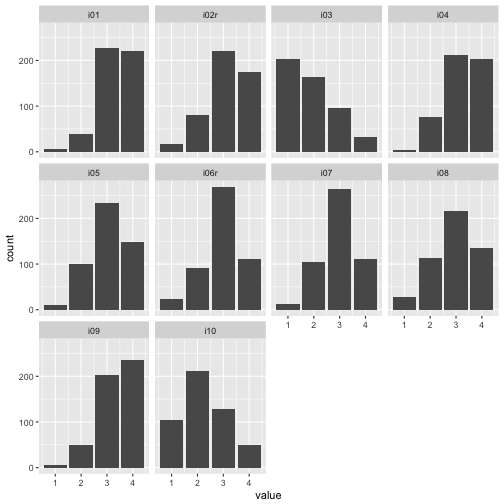Appears ok!

Ok, let’s save the data file as the last step (here, we simple chose the working directory)

write.csv(data, file = "extra.csv")


# Some thoughts (and simulation) on overfitting

Overfitting is a common problem in data analysis. Some go as far as saying that “most of” published research is false (John Ionnadis); overfitting being one, maybe central, problem of it. In this post, we explore some aspects on the notion of overfitting.

Assume we have 10 metric variables v (personality/health/behavior/gene indicator variables), and, say, 10 variables for splitting up subgroups (aged vs. young, female vs. male, etc.), so 10 dichotomic variables. Further assume there are no association whatsoever between these variables. How likely is it we find something publishable? Apparently quite probably; but let’s give it a try.

Load some packages.

library(tidyverse)
library(corrr)
library(broom)
library(htmlTable)


# Simulated dataset 10x10

Make sup some uncorrelated data, 10x10 variables each $N \sim (0,1)$.

set.seed(123)
matrix(rnorm(100), 10, 10) -> v


Let’s visualize the correlation matrix.

v %>% correlate %>% rearrange %>% rplot(print_cor = TRUE)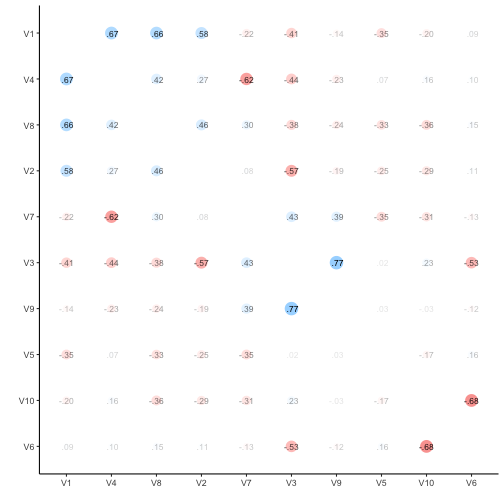v %>% correlate -> v_corr_matrix


So, quite some music in the bar. Let’s look at the distribution of the correlation coefficients.

v_corr_matrix %>%
select(-rowname) %>%
gather %>%
filter(complete.cases(.)) -> v_long

v_long %>%
mutate(r_binned = cut_width(value, 0.2)) %>%
ggplot +
aes(x = r_binned) +
geom_bar()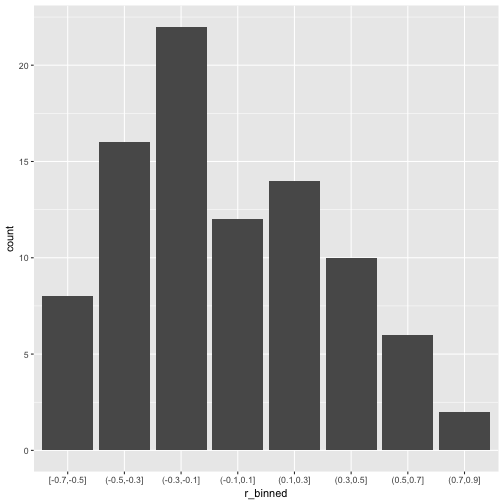What about common statistics?

library(psych)
describe(v_long$value)  ## vars n mean sd median trimmed mad min max range skew kurtosis ## X1 1 90 -0.03 0.36 -0.12 -0.05 0.35 -0.68 0.77 1.44 0.36 -0.68 ## se ## X1 0.04  Probably more stringent to just choose one triangle from the matrix, but I think it does not really matter for our purposes. Quite a bit of substantial correlation going on. Let’s look from a different angle. v_long %>% ggplot + aes(x = 1, y = value) + geom_violin(alpha = .3) + geom_jitter(aes(x = 1, y = value), width = .1) + xlab("") + geom_hline(yintercept = mean(v_long$value), linetype = "dashed", color = "#880011")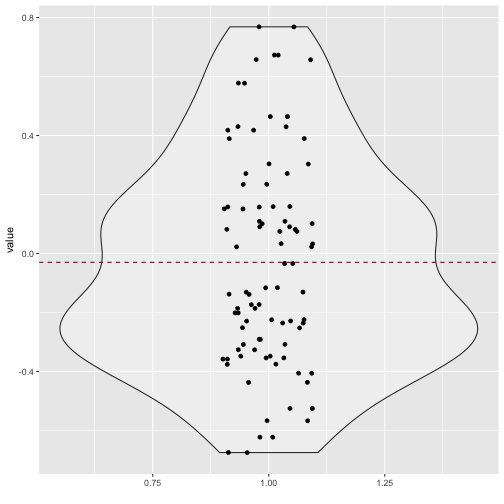## 100x100 dataset correlation

Now let’s scale up a bit and see whether the pattern stabilize.

set.seed(123)
matrix(rnorm(10^4), 100, 100) -> v2
v2 %>% correlate -> v2_corr_matrix

v2_corr_matrix %>%
select(-rowname) %>%
gather %>%
filter(complete.cases(.)) -> v2_long

v2_long %>%
mutate(r_binned = cut_width(value, 0.2)) %>%
ggplot +
aes(x = r_binned) +
geom_bar(aes(y = ..count../sum(..count..))) +
ylab("proportion")So, how many r’s are in the (0.1,0.3) bin?

library(broom)
v2_long %>%
mutate(r_binned = cut_width(value, 0.2)) %>%
group_by(r_binned) %>%
summarise(n_per_bin = n(),
prop_per_bin = round(n()/nrow(.), 2))

## # A tibble: 5 × 3
##      r_binned n_per_bin prop_per_bin
##        <fctr>     <int>        <dbl>
## 1 [-0.5,-0.3]        10         0.00
## 2 (-0.3,-0.1]      1594         0.16
## 3  (-0.1,0.1]      6776         0.68
## 4   (0.1,0.3]      1512         0.15
## 5   (0.3,0.5]         8         0.00


That’s quite a bit; 15% correlation in the bin (.1.3). Publication ahead!

# Subgroup analyses

Now, let’s look at our subgroup. For that purposes, we need actual data, not only the correlation data. Let’s come up with 1000 individuals, where he have 100 measurement (metric; N(0,1)), and 100 subgroup variables (dichotomic).

set.seed(123)
matrix(rnorm(1000*100), nrow = 1000, ncol = 100) -> v3
dim(v3)

##  1000  100

matrix(sample(x = c("A", "B"), size = 1000*100, replace = TRUE), ncol = 100, nrow = 1000) -> v4
dim(v4)

##  1000  100

v5 <- cbind(v3, v4)
dim(v5)

##  1000  200


Now, t-test battle. For each variable, compute a t-test; for each subgroup, compute a t-test. Do the whole shabeng (100*100 tests).

But, as a first step, let’s look at the first subgroup variable only.

v3 %>%
data.frame %>%
map(~t.test(.x ~ v4[, 1])) %>%
map(broom::tidy) %>%
map("p.value") %>%
unlist %>%
tibble -> v3_pvalues

v3_pvalues %>%
qplot(x = .) +
xlab("p value") +
geom_vline(xintercept = .05, color = "#880011", linetype = "dashed")

## Don't know how to automatically pick scale for object of type tbl_df/tbl/data.frame. Defaulting to continuous.

## stat_bin() using bins = 30. Pick better value with binwidth.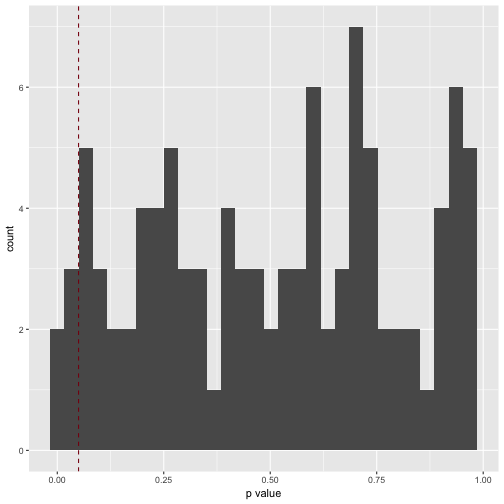How many significant p values resulted (should be 5%, ie 5 of 100)?

v3_pvalues %>%
filter(. < .05)

## # A tibble: 5 × 1
##            .
##        <dbl>
## 1 0.01350926
## 2 0.04115726
## 3 0.01064116
## 4 0.04251559
## 5 0.02505199


Which is exactly what we found.

p_list <- vector(length = 100, mode = "list")
i <- 1

for (i in 1:100){

v3 %>%
data.frame %>%
map(~t.test(.x ~ v4[, i])) %>%
map(broom::tidy) %>%
map("p.value") %>%
unlist %>%
tibble -> p_list[[i]]

}

# str(p_list)  #tl;dr

p_df <- as.data.frame(p_list)
names(p_df) <- paste("V", formatC(1:100, width = 3, flag = "0"), sep = "")


Let’s try a giant plot with 100 small multiples.

p_df %>%
gather %>%
ggplot(aes(x = value)) +
geom_histogram() +
facet_wrap(~key, ncol = 10)

## stat_bin() using bins = 30. Pick better value with binwidth.Well impressive, but that histogram matrix does not serve much. What about a correlation plot as above, only giantesque…

p_df %>% correlate %>% rplot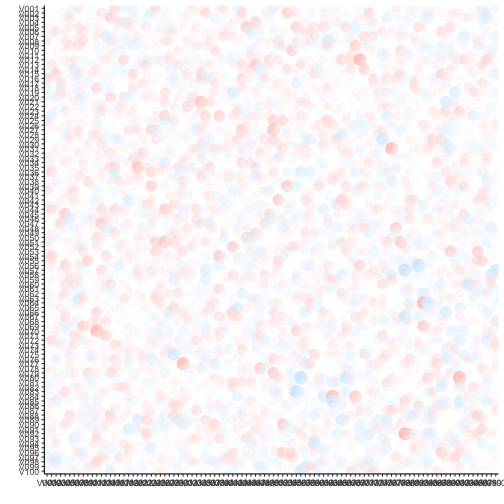Hm, of artis values (in 10000 years). Maybe better plot a histogram of the number of signifinat p-values ( <. 05).

p_df %>%
gather %>%
dplyr::filter(value < .05) %>%
qplot(x = value, data = .) +
xlab("p value")

## stat_bin() using bins = 30. Pick better value with binwidth.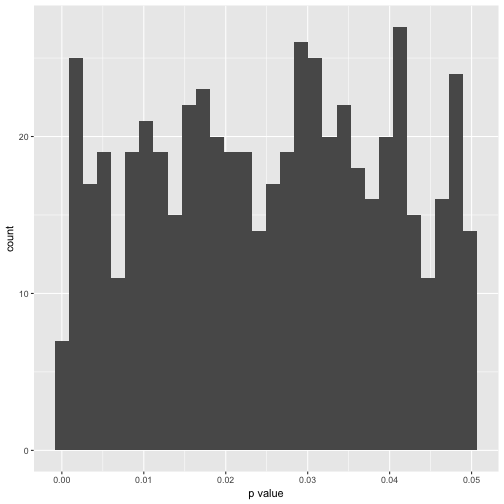In exact numbers, what’s the percentage of significant p-values per variable?

p_df %>%
gather %>%
group_by(key) %>%
filter(value < .05) %>%
summarise(n = n()) -> p_significant

htmlTable(p_significant)

key n
1 V001 5
2 V002 4
3 V003 8
4 V004 7
5 V005 4
6 V006 4
7 V007 4
8 V008 5
9 V009 3
10 V010 5
11 V011 6
12 V012 8
13 V013 7
14 V014 4
15 V015 13
16 V016 7
17 V017 8
18 V018 5
19 V019 7
20 V020 4
21 V021 10
22 V022 3
23 V023 5
24 V024 4
25 V025 7
26 V026 9
27 V027 4
28 V028 7
29 V029 5
30 V030 3
31 V031 4
32 V032 9
33 V033 8
34 V034 8
35 V035 4
36 V036 2
37 V037 11
38 V038 8
39 V039 2
40 V040 10
41 V041 6
42 V042 3
43 V043 5
44 V044 2
45 V045 8
46 V046 2
47 V047 8
48 V048 7
49 V049 2
50 V050 5
51 V051 4
52 V052 10
53 V053 5
54 V054 3
55 V055 3
56 V056 5
57 V057 3
58 V058 2
59 V059 1
60 V060 8
61 V062 6
62 V063 5
63 V064 3
64 V065 12
65 V066 4
66 V067 6
67 V068 2
68 V069 9
69 V070 6
70 V071 7
71 V072 8
72 V073 8
73 V074 8
74 V075 5
75 V076 4
76 V077 5
77 V078 4
78 V079 3
79 V080 4
80 V081 6
81 V082 9
82 V083 12
83 V084 5
84 V085 3
85 V086 6
86 V087 7
87 V088 4
88 V089 6
89 V090 6
90 V091 6
91 V092 7
92 V093 4
93 V094 5
94 V095 2
95 V096 10
96 V097 7
97 V098 6
98 V099 5
99 V100 2

Distribution of significant p-values.

p_significant %>%
qplot(x = n, data = .)

## stat_bin() using bins = 30. Pick better value with binwidth.Summary stats of significant p-values.

p_df %>%
gather %>%
group_by(key) %>%
filter(value < .05) %>%
summarise(n_p = n(),
mean_p = mean(value),
median_p = median(value),
sd_p = sd(value, na.rm = TRUE),
IQP_p = IQR(value),
min_p = min(value),
max_p = max(value)) %>%
map(mean) %>% as.data.frame -> p_summary_tab

## Warning in mean.default(.x[[i]], ...): argument is not numeric or logical:
## returning NA


Here comes the table of the mean over all 100 variables of the typical statistics.

p_summary_tab %>%
select_if(is.numeric) %>%
round %>%
htmlTable

key n_p mean_p median_p sd_p IQP_p min_p max_p
1 6 0 0 0 0 0

In sum, overfitting - mistakenly taking noise as signal - happened often. Actually, as often as is expected by theory. But, not knowing or not minding theory, one can easily be surprised by the plethora of “interesting findings” in the data. In John Tukey’s words (more or less): “Torture the data for a long enough time and it will tell you anything”.

# New bar stacking with ggplot 2.2.0

Recently, ggplot2 2.2.0 was released. Among other news, stacking bar plot was improved. Here is a short demonstration.

Load libraries

library(tidyverse)
library(htmlTable)


… and load data:

data <- read.csv("https://osf.io/meyhp/?action=download")


DOI for this piece of data is 10.17605/OSF.IO/4KGZH.

The data consists of results of a survey on extraversion and associated behavior.

Say, we would like to visualize the responsed to the extraversion items (there are 10 of them).

So, let’s see. First, compute summary of the responses.

data %>%
select(i01:i10) %>%
gather %>%
dplyr::count(key, value) %>%
ungroup -> data2

htmlTable(head(data2))

key value n
1 i01 1 7
2 i01 2 39
3 i01 3 230
4 i01 4 223
5 i01 2
6 i02 1 176

Ok, so now let’s plot.

ggplot(data2) +
geom_col(aes(x = key, fill = value, y = n))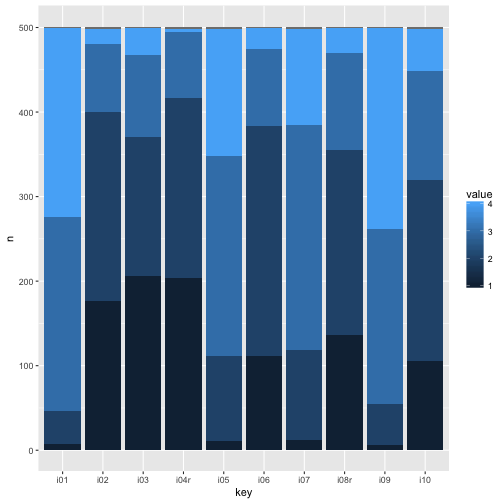Note that geom_col is short-hand vor geom_bar(stat = "identity").

Of interest, “negative” stacking is now possible.

data %>%
select(i01:i10) %>%
gather %>%
na.omit %>%
count(key, value) %>%
ungroup %>%
group_by(key) %>%
mutate(rel_n = round(n - mean(n), 2)) %>%
ungroup -> data3

htmlTable(head(data3))

key value n rel_n
1 i01 1 7 -117.75
2 i01 2 39 -85.75
3 i01 3 230 105.25
4 i01 4 223 98.25
5 i02 1 176 51.5
6 i02 2 224 99.5
ggplot(data3) +
geom_col(aes(x = key, fill = value, y = rel_n))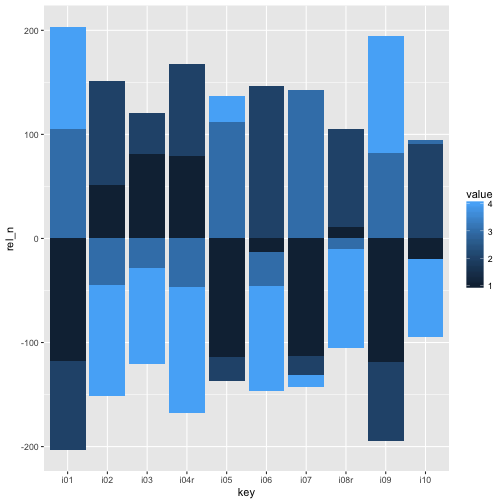Hm, at it comes to the color, we need to change to discrete colors. It appears that the color scheme is not able (out of the box) to adapt to this “negative stacking”.

So:

ggplot(data3) +
geom_col(aes(x = key, fill = factor(value), y = rel_n))sessionInfo()

## R version 3.3.2 (2016-10-31)
## Platform: x86_64-apple-darwin13.4.0 (64-bit)
## Running under: macOS Sierra 10.12.1
##
## locale:
##  en_US.UTF-8/en_US.UTF-8/en_US.UTF-8/C/en_US.UTF-8/en_US.UTF-8
##
## attached base packages:
##  stats     graphics  grDevices utils     datasets  methods   base
##
## other attached packages:
##  purrr_0.2.2.9000 readr_1.0.0      tibble_1.2       tidyverse_1.0.0
##  knitr_1.15       htmlTable_1.7    ggplot2_2.2.0    tidyr_0.6.0
##  dplyr_0.5.0
##
## loaded via a namespace (and not attached):
##   Rcpp_0.12.7         magrittr_1.5        munsell_0.4.3
##   colorspace_1.3-0    R6_2.2.0            highr_0.6
##   stringr_1.1.0       plyr_1.8.4          tools_3.3.2
##  grid_3.3.2          gtable_0.2.0        DBI_0.5-1
##  htmltools_0.3.5     yaml_2.1.13         lazyeval_0.2.0.9000
##  assertthat_0.1      digest_0.6.10       rprojroot_1.1
##  rsconnect_0.5       evaluate_0.10       rmarkdown_1.1.9016
##  labeling_0.3        stringi_1.1.2       scales_0.4.1
##  backports_1.0.4


# Crashkurs zur Erstellung von Barplots für Umfrage-Daten

Eine recht häufige Art von Daten in der Wirtschaft kommen von Umfragen in der Belegschaft. Diese Daten gilt es dann aufzubereiten und graphisch wiederzugeben. Dafür gibt dieser Post einige grundlegende Hinweise. Grundwissen mit R setzen wir voraus :-)

Eine ausführlichere Beschreibung hier sich z.B. hier.

# Packages laden

Nicht vergessen: Ein Computerprogramm (z.B. ein R-Package) kann man nur dann laden, wenn man es vorher installier hat (aber es reicht, das Programm/R-Package einmal zu installieren).

Im Paket tidyverse sind eine Reihe von Paketen gebündelt, die wir brauchen z.B. zum Diagramme erstellen (ggplot2) oder um Daten zu verhackstücken (dplyr, tidyr).

library(tidyverse)


# Daten einlesen

Jetzt lesen wir die Daten ein. In RStudio gibt es dafür z.B. den Button “Import Dataset”; der R-Commander hat einen ähnlichen Menüpunkt. Auch Excel-Dateien kann man so einlesen.

Hier laden wir mal beispielhaft einen Datensatz zu einer Online-Umfrage:

data <- read.csv("https://osf.io/meyhp/?action=download")


Der DOI für diesen Datensatz ist 10.17605/OSF.IO/4KGZH.

Der Datensatz besteht aus 10 Extraversions-Items (B5T nach Satow) sowie einigen Verhaltenskorrelaten (zumindest angenommenen). Uns interessieren also hier nur die 10 Extraversions-Items, die zusammen Extraversion als Persönlichkeitseigenschaft messen (sollen). Wir werden die Antworte der Befragten darstelle, aber uns hier keine Gedanken über Messqualität u.a. machen.

Die Umfrage kann hier eingesehen werden.

Schauen wir uns die Daten mal an:

glimpse(data)

## Observations: 501
## Variables: 27
## $timestamp <fctr> 11.03.2015 19:17:48, 11.03.2015 19:18:05, ... ##$ code               <fctr> HSC, ERB, ADP, KHB, PTG, ABL, ber, hph, IH...
## $i01 <int> 3, 2, 3, 3, 4, 3, 4, 3, 4, 4, 3, 3, 4, 4, 3... ##$ i02                <int> 2, 3, 1, 2, 2, 3, 1, 2, 1, 1, 2, 1, 2, 2, 2...
## $i03 <int> 3, 1, 1, 2, 1, 1, 1, 2, 1, 2, 1, 1, 1, 4, 1... ##$ i04r               <int> 2, 3, 1, 1, 1, 1, 2, 2, 1, 1, 2, 2, 3, 1, 2...
## $i05 <int> 4, 3, 4, 3, 4, 2, 3, 2, 3, 3, 3, 2, 3, 3, 3... ##$ i06                <int> 1, 3, 4, 2, 2, 2, 2, 3, 1, 2, 2, 2, 2, 2, 2...
## $i07 <int> 3, 2, 3, 3, 4, 4, 2, 3, 3, 3, 2, 4, 2, 3, 3... ##$ i08r               <int> 3, 2, 3, 2, 3, 2, 2, 3, 2, 2, 2, 3, 2, 2, 1...
## $i09 <int> 3, 3, 3, 3, 3, 3, 3, 4, 4, 3, 4, 2, 4, 4, 4... ##$ i10                <int> 1, 1, 1, 2, 4, 3, 2, 1, 2, 3, 1, 3, 2, 3, 2...
## $n_facebook_friends <int> 250, 106, 215, 200, 100, 376, 180, 432, 200... ##$ n_hangover         <dbl> 1, 0, 0, 15, 0, 1, 1, 2, 5, 0, 1, 2, 20, 2,...
## $age <int> 24, 35, 25, 39, 29, 33, 24, 28, 29, 38, 25,... ##$ sex                <fctr> Frau, Frau, Frau, Frau, Frau, Mann, Frau, ...
## $extra_single_item <int> 4, 3, 4, 3, 4, 4, 3, 3, 4, 4, 4, 4, 4, 4, 4... ##$ time_conversation  <dbl> 10, 15, 15, 5, 5, 20, 2, 15, 10, 10, 1, 5, ...
## $presentation <fctr> nein, nein, nein, nein, nein, ja, ja, ja, ... ##$ n_party            <dbl> 20, 5, 3, 25, 4, 4, 3, 6, 12, 5, 10, 5, 10,...
## $clients <fctr> , , , , , , , , , , , , , , , , , , , , , ... ##$ extra_vignette     <fctr> , , , , , , , , , , , , , , , , , , , , , ...
## $extra_description <int> NA, NA, NA, NA, NA, NA, NA, NA, NA, NA, NA,... ##$ prop_na_per_row    <dbl> 0.04347826, 0.04347826, 0.04347826, 0.04347...
## $extra_mean <dbl> 2.5, 2.3, 2.4, 2.3, 2.8, 2.4, 2.2, 2.5, 2.2... ##$ extra_median       <dbl> 3.0, 2.5, 3.0, 2.0, 3.0, 2.5, 2.0, 2.5, 2.0...
## $n_clients <dbl> NA, NA, NA, NA, NA, NA, NA, NA, NA, NA, NA,...  # Daten aufbereiten Daten aufbereiten ist ein umfangreiches Geschäft; hier schneiden wir diese Angelegenheit nur kurz an. Man sollte (u.a.): • fehlende Werte identifizieren, evtl. die Fälle löschen, imputieren oder … nix tun • möglich Fehleingaben identifizieren • Negativ gepolte Items unkodieren • Mittelwerte bilden Statt dessen gehen wir davon aus, dass alles passt :-) Eine ausführlichere Beschreibung der Aufbereitung dieser Daten findet sich hier. # Daten umstellen für das Plotten Wir haben ein Diagramm vor Augen (s.u.), bei dem auf der X-Achse die Items stehen (1,2,…,n) und auf der Y-Achse die Anzahl der Kreuze nach Kategorien. Viele Grafik-Funktionen sind nun so aufgebaut, dass auf der X-Achsen nur eine Variable steht. ggplot2, das wir hier verwenden, ist da keine Ausnahme. Wir müssen also die “breite” Tabelle (10 Spalten, pro Item eine) in eine “lange Spalte” umbauen: Eine Spalte heißt dann “Itemnummer” und die zweite “Wert des Items” oder so ähnlich. Eine ausführliche Beschreibung findet sich z.B. hier. Also, los geht’s: Zuerst wählen wir aus der Fülle der Daten, die Spalten, die uns interessieren: Die 10 Extraversions-Items, in diesem Fall. data_items <- select(data, i01:i10)  Dann stellen wir die Daten von “breit” nach “lang” um, so dass die Items eine Variable bilden und damit für ggplot2 gut zu verarbeiten sind. data_long <- gather(data_items, key = items, value = Antwort) data_long$Antwort <- factor(data_long\$Antwort)


Den Befehl mit factor brauchen wir für zum Diagramm erstellen im Folgenden. Dieser Befehl macht aus den Zahlen bei der Variable Antwort eine nominale Variable (in R: factor) mit Text-Werten “1”, “2” und so weiter. Wozu brauchen wir das? Der Digrammbefehl unten kann nur mit nominalen Variablen Gruppierungen durchführen. Wir werden in dem Diagramm die Anzahl der Antworten darstellen - die Anzahl der Antworten nach Antwort-Gruppe (Gruppe mit Antwort “1” etc.).

Keine Sorge, wenn sich das reichlich ungewöhnlich anhört. Sie müssen es an dieser Stelle nicht erfinden :-)

Man gewöhnt sich daran einerseits; und andererseits ist es vielleicht auch so, dass diese Funktionen nicht perfekt sind, oder nicht aus unserer Sicht oder nur aus Sicht des Menschen, der die Funktion geschrieben hat. Jedenfalls brauchen wir hier eine factor Variable zur Gruppierung…

Damit haben wir es schon! Jetzt wird gemalt.

# Erste Diagramme

Wir nutzen ggplot2, wie gesagt, und davon die Funktion qplot (q wie quick, nehme ich an.).

ggplot(data = data_long) +
aes(x = items)  +
geom_bar(aes(fill = Antwort), position = "fill")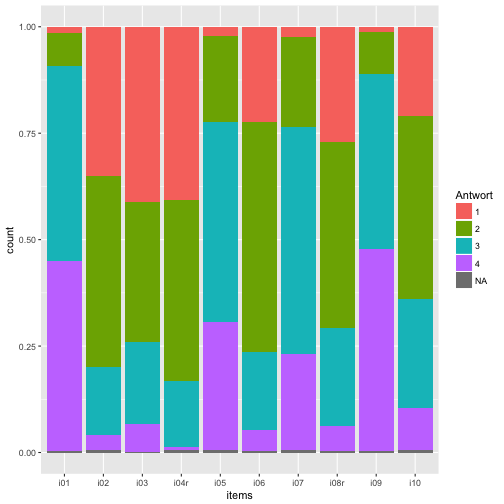Was macht dieser ggplot Befehl? Schauen wir es uns in Einzelnen an:

• ggplot(data = ...): Wir sagen “Ich möchte gern die Funktion ggplot nutzen, um den Datensatz … zu plotten”.
• aes(...): Hier definieren wir die “aesthetics” des Diagramms, d.h. alles “Sichtbare”. Wir ordnen in diesem Fall der X-Achse die Variable items zu. Per Standardeinstellung geht ggplot davon aus, dass sie die Häufigkeiten der X-Werte auf der Y-Achse haben wollen, wenn Sie nichts über die Y-Achse sagen. Jetzt haben wir ein Koordinatensystem definiert (das noch leer ist).
• geom_bar(): “Hey R oder ggplot, jetzt male mal einen barplot in den ansonsten noch leeren plot”.
• aes(fill = Antwort): Genauer gesagt nutzen wir aes um einen sichtbaren Aspekte des Diagramms (wie die X-Achse) eine Variable des Datensatzes zuzuordnen. Jetzt sagen wir, dass die Füllung (im Balkendiagramm) durch die Werte von Antwort definiert sein sollen (also “1”, “2” etc.).
• position = "fill" sagt, dass die Gesamt-Höhe des Balken aufgeteilt werden soll mit den “Teil-Höhen” der Gruppen (Antwort-Kategorien 1 bis 4); wir hätten die Teil-Höhen auch nebeneinander stellen können.

# Nas entfernen

Vielleicht ist es schöner, die NAs erst zu entfernen.

data_long <- na.omit(data_long)


Und dann noch mal plotten:

ggplot(data = data_long) +
aes(x = items)  +
geom_bar(aes(fill = Antwort), position = "fill")# Um 90° drehen

Dazu nehmen wir + coord_flip(), also “flippe das Koordinatensystem”.

ggplot(data = data_long) +
aes(x = items)  +
geom_bar(aes(fill = Antwort), position = "fill") +
coord_flip()# Text-Labels für die Items

Wir definieren die Texte (“Labels”) für die Items:

item_labels <- c("Ich bin das erste Item",
"Das zweite Item",
"Item 3 sdjfkladsjk",
"Ich bin ein krasser Couch-Potato UMKODIERT",
"i5 asf", "i6 sdf", "adfjks", "sfjlkd", "sdfkjl", "sdfjkl")


Jetzt hängen wir die Labels an die Items im Diagramm:

ggplot(data = data_long) +
aes(x = items)  +
geom_bar(aes(fill = Antwort), position = "fill") +
coord_flip() +
scale_x_discrete(labels = item_labels)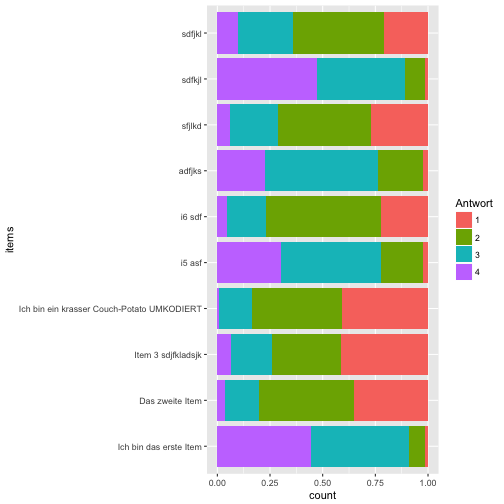Man kann auch einen Zeilenumbruch in den Item-Labels erzwingen… wobei das führt uns schon recht weit, aber gut, zum Abschluss :-)

item_labels <- c("Ich bin das erste Item",
"Das zweite Item",
"Item 3 sdjfkladsjk",
"Ich bin ein krasser \nCouch-Potato***mit Zeilenumbruch***",
"i5 asf", "i6 sdf", "adfjks", "sfjlkd", "sdfkjl", "sdfjkl")


Und wieder plotten:

ggplot(data = data_long) +
aes(x = items)  +
geom_bar(aes(fill = Antwort), position = "fill") +
coord_flip() +
scale_x_discrete(labels = item_labels, name = "Extraversionsitems") +
scale_y_continuous(name = "Anteile")# Diagramm mit Häufigkeiten, nicht Anteilen

Ach so, schön wäre noch die echten Zahlen an der Y-Achse, nicht Anteile. Dafür müssen wir unseren Diagrammtyp ändern, bzw. die Art der Anordnung ändern. Mit position = "fill" wird der Anteil (also mit einer Summe von 100%) dargestellt. Wir können auch einfach die Zahlen/Häufigkeiten anzeigen, in dem wir die Kategorien “aufeinander stapeln”

ggplot(data = data_long) +
aes(x = items)  +
geom_bar(aes(fill = Antwort), position = "stack") +
coord_flip() +
scale_x_discrete(labels = item_labels)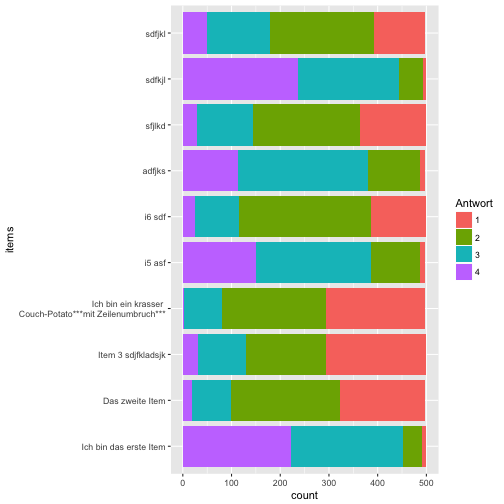# Farbschema

Ja, die Wünsche hören nicht auf… Also, noch ein anderes Farbschema:

ggplot(data = data_long) +
aes(x = items)  +
geom_bar(aes(fill = Antwort), position = "stack") +
coord_flip() +
scale_x_discrete(labels = item_labels) +
scale_fill_brewer(palette = 17)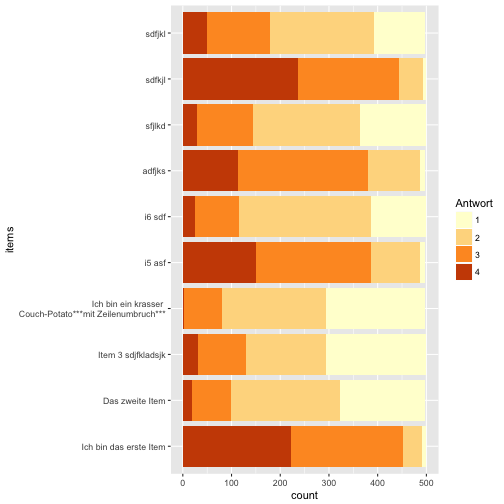Probieren Sie mal ein paar Brewer Farbpaletten aus.

Natürlich kann man noch viel mehr tun; schauen Sie sich z.B. dieses ausführlichere Posting an: https://sebastiansauer.github.io/plotting_surveys/.